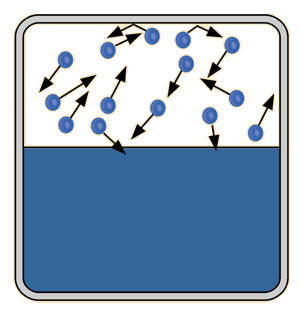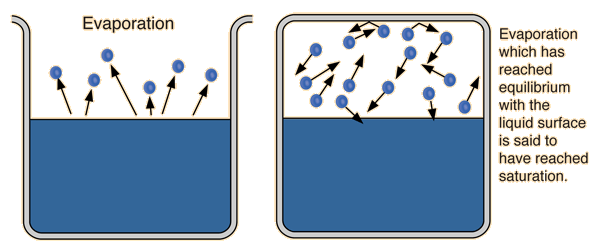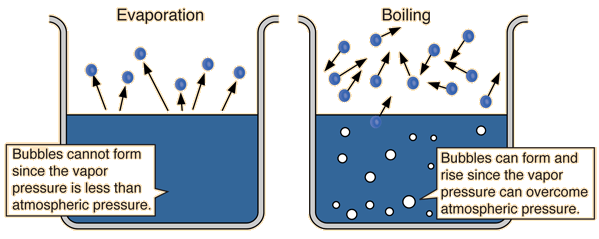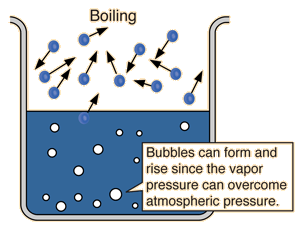# Saturated Vapor Pressure

The process of evaporation in a closed container will proceed until there are as many molecules returning to the liquid as there are escaping. At this point the vapor is said to be saturated, and the pressure of that vapor (usually expressed in mmHg) is called the saturated vapor pressure.
 Since the molecular kinetic energy is greater at higher temperature, more molecules can escape the surface and the saturated vapor pressure is correspondingly higher. If the liquid is open to the air, then the vapor pressure is seen as a partial pressure along with the other constituents of the air. The temperature at which the vapor pressure is equal to the atmospheric pressure is called the boiling point.Table for water Graph for water
Index

Kinetic theory concepts

Applications of kinetic theory

Vapor application concepts

 HyperPhysics***** Thermodynamics R Nave
Go Back

# Evaporation

Ordinary evaporation is a surface phenomenon - some molecules have enough kinetic energy to escape. If the container is closed, an equilibrium is reached where an equal number of molecules return to the surface. The pressure of this equilibrium is called the saturation vapor pressure.In order to evaporate, a mass of water must collect the large heat of vaporization, so evaporation is a potent cooling mechanism. Evaporation heat loss is a major climatic factor and is crucial in the cooling of the human body.
 Saturation vapor pressure Difference between evaporation and boiling
Index

Kinetic theory concepts

Applications of kinetic theory

Vapor application concepts

 HyperPhysics***** Thermodynamics R Nave
Go Back

# Evaporation vs Boiling

Ordinary evaporation is a surface phenomenon - since the vapor pressure is low and since the pressure inside the liquid is equal to atmospheric pressure plus the liquid pressure, bubbles of water vapor cannot form. But at the boiling point, the saturated vapor pressure is equal to atmospheric pressure, bubbles form, and the vaporization becomes a volume phenomenon.Index

Kinetic theory concepts

Applications of kinetic theory

Vapor application concepts

 HyperPhysics***** Thermodynamics R Nave
Go Back

# Boiling Point

The boiling point is defined as the temperature at which the saturated vapor pressure of a liquid is equal to the surrounding atmospheric pressure. For water, the vapor pressure reaches the standard sea level atmospheric pressure of 760 mmHg at 100°C. Since the vapor pressure increases with temperature, it follows that for pressure greater than 760 mmHg (e.g., in a pressure cooker), the boiling point is above 100°C and for pressure less than 760 mmHg (e.g., at altitudes above sea level), the boiling point will be lower than 100°C. As long as a vessel of water is boiling at 760 mmHg, it will remain at 100°C until the phase change is complete. Rapidly boiling water is not at a higher temperature than slowly boiling water. The stability of the boiling point makes it a convenient calibration temperature for temperature scales.### At the boiling point, saturated vapor pressure equals atmospheric pressure.

 Boiling and evaporation Boiling point variation with pressure
 Boiling point elevation by dissolved matter
Index

Kinetic theory concepts

Applications of kinetic theory

Vapor application concepts

 HyperPhysics***** Thermodynamics R Nave
Go Back

# Boiling Point Variation

The standard boiling point for water at 100°C is for standard atmospheric pressure, 760 mmHg. It is the experience of high altitude hikers that it takes longer to cook food at altitude because the boiling point of water is lower. On the other hand, food cooks more quickly in a pressure cooker because the boiling point is elevated. Raising or lowering the pressure by about 28 mmHg will change the boiling point by 1°C.

Although the vapor pressure variation with temperature is a non-linear one, the boiling point variation can be approximated near 100°C by an empirical fit of the available data. This can provide the following estimate of the boiling point:

For a pressure of mmHg
the boiling point will be approximately °C.

For variations in atmospheric pressure with altitude according to the barometric formula, the boiling point at a height of m = ft above sea level (atmos. pressure mmHg)
would be approximately °C.

### Any of the data values above may be changed. The empirical calculation is valid only for a few degrees above and below the normal boiling point.

Notes: This calculation assumes an air temperature of 20°C and an average air molecule mass of 29 amu. The atmospheric pressure drops off a little faster with height when the air is colder, but the change is not large over the range of reasonable daytime temperatures. If you put in pressures above atmospheric pressure, you get reasonable boiling point values so long as you are within a few degrees of normal, but the negative altitude values you get are not valid.
 Barometric formula
 Applications of increased pressure Pressure Cooker Auto Cooling System
Index

Kinetic theory concepts

Applications of kinetic theory

Vapor application concepts

 HyperPhysics***** Thermodynamics R Nave
Go Back

# Boiling Point Variation Near 100 CValues were taken from the saturated vapor pressure table for water near 100 degrees Celsius. An empirical fit to these data values was made, and the formula obtained is shown on the diagram. It could be considered to be reasonably valid only for a few degrees above and below 100 °C since the curve is very non-linear.

 Calculation based on this curve fit
Index

Kinetic theory concepts

Applications of kinetic theory

Vapor application concepts

 HyperPhysics***** Thermodynamics R Nave
Go Back